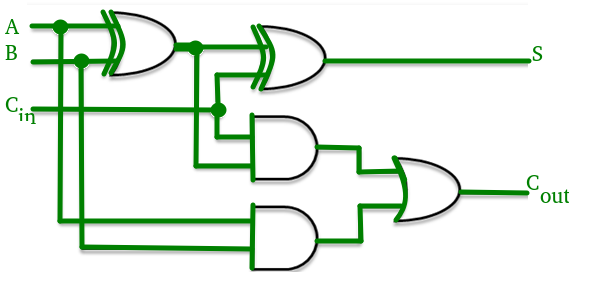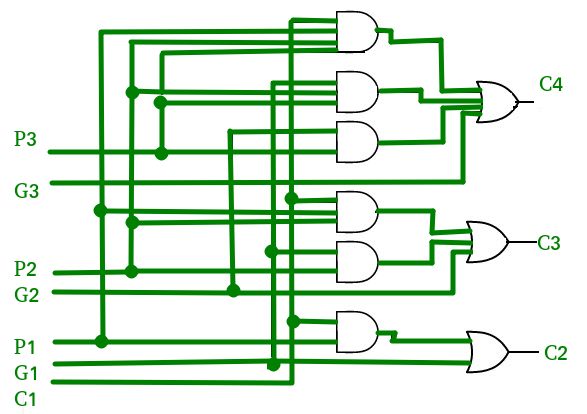GeeksforGeeks App
Open AppBrowser
Continue

## Related Articles

The adder produce carry propagation delay while performing other arithmetic operations like multiplication and divisions as it uses several additions or subtraction steps. This is a major problem for the adder and hence improving the speed of addition will improve the speed of all other arithmetic operations. Hence reducing the carry propagation delay of adders is of great importance. There are different logic design approaches that have been employed to overcome the carry propagation problem. One widely used approach is to employ a carry look-ahead which solves this problem by calculating the carry signals in advance, based on the input signals. This type of adder circuit is called a carry look-ahead adder.

Here a carry signal will be generated in two cases:

1. Input bits A and B are 1
2. When one of the two bits is 1 and the carry-in is 1.

In ripple carry adders, for each adder block, the two bits that are to be added are available instantly. However, each adder block waits for the carry to arrive from its previous block. So, it is not possible to generate the sum and carry of any block until the input carry is known. Theblock waits for theblock to produce its carry. So there will be a considerable time delay which is carry propagation delay.Consider the above 4-bit ripple carry adder. The sumis produced by the corresponding full adder as soon as the input signals are applied to it. But the carry inputis not available on its final steady-state value until carryis available at its steady-state value. Similarlydepends onandon. Therefore, though the carry must propagate to all the stages in order that outputand carrysettle their final steady-state value.

The propagation time is equal to the propagation delay of each adder block, multiplied by the number of adder blocks in the circuit. For example, if each full adder stage has a propagation delay of 20 nanoseconds, thenwill reach its final correct value after 60 (20 × 3) nanoseconds. The situation gets worse, if we extend the number of stages for adding more number of bits.

A carry look-ahead adder reduces the propagation delay by introducing more complex hardware. In this design, the ripple carry design is suitably transformed such that the carry logic over fixed groups of bits of the adder is reduced to two-level logic. Let us discuss the design in detail.Consider the full adder circuit shown above with corresponding truth table. We define two variables as ‘carry generate’and ‘carry propagate’then,The sum output and carry output can be expressed in terms of carry generateand carry propagateaswhereproduces the carry when bothare 1 regardless of the input carry.is associated with the propagation of carry fromtoThe carry output Boolean function of each stage in a 4 stage carry look-ahead adder can be expressed asFrom the above Boolean equations we can observe thatdoes not have to wait forandto propagate but actuallyis propagated at the same time asand. Since the Boolean expression for each carry output is the sum of products so these can be implemented with one level of AND gates followed by an OR gate.

The implementation of three Boolean functions for each carry output (and) for a carry look-ahead carry generator shown in below figure.Time Complexity Analysis :

1. The part that computes the carry for each bit.
2. The part that adds the input bits and the carry for each bit position.

Thecomplexity arises from the part that generates the carry, not the circuit that adds the bits.
Now, for the generation of thecarry bit, we need to perform a AND between (n+1) inputs. The complexity of the adder comes down to how we perform this AND operation. If we have AND gates, each with a fan-in (number of inputs accepted) of k, then we can find the AND of all the bits intime. This is represented in asymptotic notation as• The propagation delay is reduced.
• It provides the fastest addition logic.

• The Carry Look-ahead adder circuit gets complicated as the number of variables increase.
• The circuit is costlier as it involves more number of hardware.

NOTE :

For n-bit carry lookahead adder to evaluate all the carry bits it requires [n(n + 1)]/2 AND gates and n OR gates.

GATE CS Corner Questions

Practicing the following questions will help you test your knowledge. All questions have been asked in GATE in previous years or in GATE Mock Tests. It is highly recommended that you practice them.

My Personal Notes arrow_drop_up